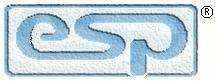Elliott Sound Products Measuring Loudspeaker Parameters

Measuring Loudspeaker Parameters

© 2000 - Rod Elliott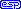Updated June 2018Articles IndexMain Index

Contents
1   Measuring Thiele / Small Loudspeaker Parameters

There are several different ways to measure the Thiele/Small parameters of a loudspeaker driver.  The method described here provides a way for the beginner and DIY enthusiast to measure the parameters without any expensive or specialised equipment.  While every care is taken to ensure that calculations and formulae are correct, ESP accepts no liability for errors or omissions.

Definitions:

 Re Electrical resistance of voice coil Fs Resonant frequency of loudspeaker moving mass (in free air) Qes Electrical Q of loudspeaker Qms Mechanical Q of loudspeaker Qts Total Q of loudspeaker Vas Equivalent air volume of moving mass suspension

1.1   Measuring Re, Fs, Qes, Qms and Qts

To measure these parameters using the method outlined below, you'll need to have the following items:

• A power amplifier, rated at 1-10 Watts (RMS) or thereabouts (must have low output impedance <0.1 ohm)
• Audio frequency oscillator (PC based is fine)
• Digital multimeter (with frequency measurement), or PC based instrument
• An accurate test resistor (any value, although I suggest 10 ohms) A ½W component will be quite sufficient.
• Alligator clip leads - you will need 4 sets of leads (leads may be soldered instead if desired)

Figure 1 shows a typical impedance curve for a loudspeaker (see Figure 5 for the equivalent circuit of this speaker, which was simulated for this article).  Resonance causes a large increase in impedance, and at some higher frequency, the inductance (or semi-inductance) of the voice coil causes the impedance to rise again.  The region for the initial measurements must be within the 'linear' region of the impedance curve.  In the example below, resonance is at 27Hz, and the linear region ranges from about 100Hz to 400Hz.

At resonance, the speaker impedance is pure resistance.  As the frequency increases towards resonance, the impedance characteristic is inductive.  Beyond resonance as impedance falls, the impedance characteristic is capacitive.  Within the 'linear' region, the impedance is again (almost) resistive, but at slightly below the speaker's nominal impedance (nominal impedance is usually taken as an average value over the usable frequency range).  At the frequency where the inductance of the voice coil becomes significant, impedance rises, and is progressively more inductive as the frequency rises.  It is common to add a compensation network to maintain an overall resistive characteristic at these higher frequencies, so that the performance of the (passive) crossover network is not compromised.  This is not necessary with an active crossover.

Although a 'pure' inductance is shown in the equivalent circuit, this component is often referred to as 'semi-inductance'.  Because of losses (primarily eddy current losses within the pole pieces), the impedance typically rises at around 3-4dB/ octave, rather than the expected (and simulated) 6dB/ octave.  This has little or no effect on resonance parameters, and can usually be ignored for these measurements.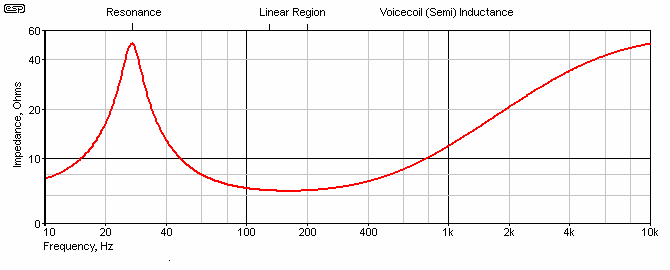Figure 1 - Loudspeaker Impedance Curve

The multimeter should be capable of measuring frequency, as well as AC voltage and resistance.  If it cannot, a frequency counter is highly recommended, since the frequency measurements are critical.  The amplifier must be capable of reproducing from 10 Hz to 2 kHz with no variation in output voltage.  It is imperative that it is insensitive to any load above 4 ohms.  The audio oscillator must also produce a signal with relatively low distortion, and the output voltage must not vary as the frequency is adjusted.  If a PC signal generator is used, it will usually display the frequency fairly accurately, but you still need to verify that output level is constant with frequency.  Many PC instruments are incapable of fractional frequencies, which may limit the accuracy of the final result.

The need for accuracy cannot be stressed too highly if accurate parameters are expected, but this is subject to reality.  It must be understood that there are many variables and many opportunities for things to go awry - during measurement, construction and normal operation.  Loudspeakers are variable beasties at best, and 'perfect' results will never be obtained in practice.  The room will usually cause more and greater errors than a small measurement error here.  While getting accurate T/S parameters is obviously important, they may be different for apparently identical drivers, and they will also change with atmospheric conditions.

Measure the resistance across the speaker terminals to obtain Re

Measure the exact resistance of the 10 ohm source resistor, Rs

The loudspeaker driver should be suspended in free space, with no obstructions or interfering surfaces nearby.  Any boundary closer than around 600mm (about 2ft) will affect the accuracy of the measurements

Connect the circuit as shown in Figure 2, and set the oscillator to approximately 100 to 200 Hz (or around 2-3 octaves above resonance) - it must be within the 'linear' range as shown on the graph above.

Set the output of the amplifier to between 0.5V and 1.0V (this is Vs).  Check that the speaker is nowhere near resonance, by changing the oscillator frequency by 50Hz or so in either direction, and measure the voltage across the resistor.  It should not change by any appreciable amount.

When you have set Vs to 0.5-1V, Is (reference speaker current) equals the meter reading (at 200Hz or other frequency as described) divided by the value of Rs.  You are measuring the voltage across the test resistor to calculate voicecoil current.

You may need to try different voltages, depending on the accuracy of your readings (or calculations).  Do not be tempted to use a voltage any higher than around 1V RMS , as the speaker may be driven outside its linear range, which ruins the validity of the measurements.  The parameters being measured are 'small signal', and it essential that a small signal is actually used.  With an 8 Ohm driver, 10 Ohm resistor and 1V signal, you will typically have a nominal current of around 55mA.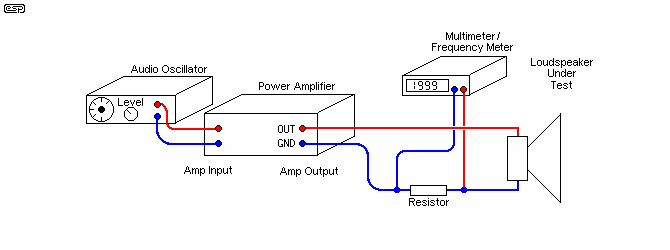Figure 2 - Measuring Speaker Parameters

The traditional way to measure Q is to measure the bandwidth between the -3dB frequencies, then divide the resonant frequency by the bandwidth.  For example, if resonance is at 29.6Hz and -3dB frequencies are at 25Hz and 35Hz, then Q is 2.96.  This would be Qms in the calculations.  This method may be suitable for low-Q drivers, but you can easily make a tiny error (causing a large final calculation error) with high-Q drivers.

In Small's original paper, f1 and f2 are the frequencies where the drive unit impedance is √( r0 ) × Re.  Likewise, r0 = ( Re + Res) / Re, (Re + Res) being the impedance at fs.  He chose √( r0 ) × Re because this simplified the calculations for Qms and Qes.

Many of the methods described elsewhere rely on a more complex formula that uses -6dB or even -9dB as the reference point to determine Q.  This makes the measurement accuracy slightly less critical.  The method below describes the -6dB method, which gives a reasonable compromise between ease of measurement and accuracy.

First, measure the resonant frequency.  Adjust the frequency until the voltage across the resistor reaches a null (minimum level).  Without changing anything, carefully measure the frequency and voltage across the resistor ...

 Frequency Fs Voltage across the resistor Vm

Calculate the following ...

 Speaker current Im = Vm / Rs Resonance impedance Rm = (Vs - Vm) / Im r0 (reference value) r0 = Is / Im -6dB current Ir = √(Im × Is) -6dB voltage Vr = Ir × Rs

Complete the measurements for Fl and Fh, for which the voltage across the source resistor is equal to Vr, and as a sanity check (to ensure that your calculations and measurements are accurate), calculate the resonant frequency based on these last two measurements.  Note that these measurements are critical, and even a small error will cause large deviations in the driver parameters.

 Check that ... √(Fl × Fh) = Fs

If the above checks out (within 1Hz or less), then Qes, Qms and Qts can be calculated as follows ...

 Mechanical Q Qms = Fs × √r0 / (Fh - Fl) Electrical Q Qes = Qms / (r0 - 1)     See note below Electrical Q Qes = (Qms / (r0 - 1)) × (Re / (Rs + Re)) Total Q Qts = Qms × Qes / (Qms + Qes)

Note:   While this is supposed to be the correct formula, many have found it gives Qes that's much too high.

You can use the spreadsheet to perform the calculations automatically for you: ls-param.xls

2   Measuring Vas (equivalent air compliance), Method 1

There are two methods for determining Vas.  The first is a known box, and the procedure is as follows ...

To measure Vas, use a good solid enclosure of known volume that is approximately a cube of the nominal speaker size.  For example, a 300mm driver (12") needs a box of about 28 litres (1 cu ft).  For reference, a cubic foot is 28.3168 litres, and one litre is contained by a cube of 100mm (10cm) to each side.

HINT:   If you make all measurements in centimetres, the result will be in millilitres (cubic centimetres, or cc).  This makes it very easy to convert to litres ... simply divide by 1,000.  If you work in millimetres (mm), the result is less intuitive, although you can still get to litres by dividing by 1,000,000.    (For those who insist on using outmoded measurement systems, I can provide a spreadsheet that uses cubits as the base linear measurement, and firkins for volume.  I will part with this upon receipt of a kilderkin of Australian \$2 coins or its equivalent in gold bullion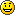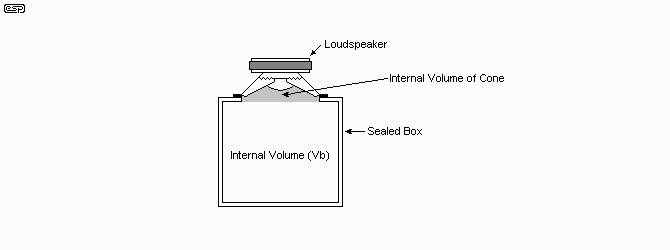Figure 3 - Setup for Measuring Vas

Determine the total volume, including the speaker cut-out and that trapped by the cone with the speaker mounted on the outside of the box for easy access.  Measure the resonant frequency in this situation, and use the free air space resonant frequency determined as shown above.  Determining the volume trapped by the speaker cone is slightly tricky.

Use one of the following methods ...

1. Place the driver in a plastic bag, ensuring it is completely sealed.  The bag should be loose enough so that it can be pushed easily into the cone area.  Place the wrapped speaker on a flat surface, with the cone facing upwards.  The cone may now safely be filled with grain (such as rice, wheat, etc), and the grain carefully poured out into a measuring jug.  The resulting measurement will be a little greater than the actual volume because the cone will be depressed by the mass of the grain.  The area of the speaker cutout in the cabinet must still be added.  Don't omit the plastic bag, as fine dust may penetrate the speaker without it.

2. Take a series of measurements.  The internal cone area is measured, then divided into sections whose volume may be calculated.  For most speakers we will have two basic shapes to deal with, and although this method is not 100% accurate, it will probably give a more than acceptable result in the majority of cases.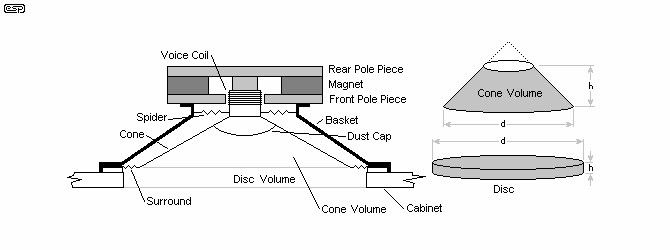Figure 4 - Determining the Volume of the Cone

There is a flat cylinder (disc) that is formed by the outer area of the basket and the cutout in the enclosure.  While there is a small error by just assuming that the cone extends fully (rather than being truncated by the dust cap), the error will generally be small.  Since loudspeaker parameters change with time anyway, the error will normally be sufficiently small as to not be an issue.  Feel free to measure the cone volume using the alternate method if it makes you feel better.

The volume of the disc is given by the conventional formula ...

Vdisc = π × r² × h    (where r is the radius and h is height)

The cone's volume is given by ...

Vcone = ( π × r² × h ) / 3

Note that the disc volume may extend to the speaker surround, and the cone diameter may be smaller than the cutout.  Make sure you measure both diameters and use the correct measurement for each calculation (as shown in the drawing above).

The total speaker volume is simply the sum of the two volumes calculated above.  Box volume is calculated as one normally would, taking great care to ensure that the measurements are accurate.  The box may be braced, but must have no fibreglass or other sound deadening material inside.  Make sure that the volume occupied by any bracing is accounted for in your calculations.  Even a simple box will be sufficiently rigid at the frequencies of interest, so a completely acoustically dead cabinet is not required (although it won't hurt).  Do not use any speaker cabinet filling material for this test.

Vas = Vb × (( Fb / Fs )² - 1 )

where Vb is the volume trapped by the speaker and box, and Fb is the resonance frequency of speaker and box combined.  Fs is the free air resonance measured previously.

2.1   An Example Calculation

A dummy test loudspeaker was used to demonstrate the process, and I have used a simulation of this speaker in the calculations shown.  The equivalent circuit is shown in Figure 5.  This circuit was also used to create the impedance graph shown in Figure 1.  It does not represent any particular driver.  However, the equivalent circuit is applicable to almost all speaker drivers, and only the values change.  The spreadsheet calculates the approximate values, and these will be useful if you need to design an impedance compensation network.  The voicecoil inductance (or semi-inductance) is not calculated because measurements aren't taken at the frequencies where it becomes significant.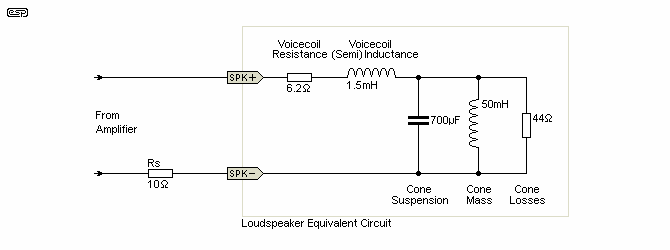Figure 5 - Dummy Test Loudspeaker

The following screen shot shows the values for the speaker, and the only contrived (i.e. invented) value is for the resonance in the sealed box.  It was necessary to invent a number here, as it is not possible to simulate it.  The final figure shown is fairly typical of many such drivers, so is not too far from the truth either.  Note that the equivalent circuit (R (losses), L (mass) & C (suspension)) of the driver at resonance is calculated by the spreadsheet.  These are shown in the 'Reference Data' section (bottom right).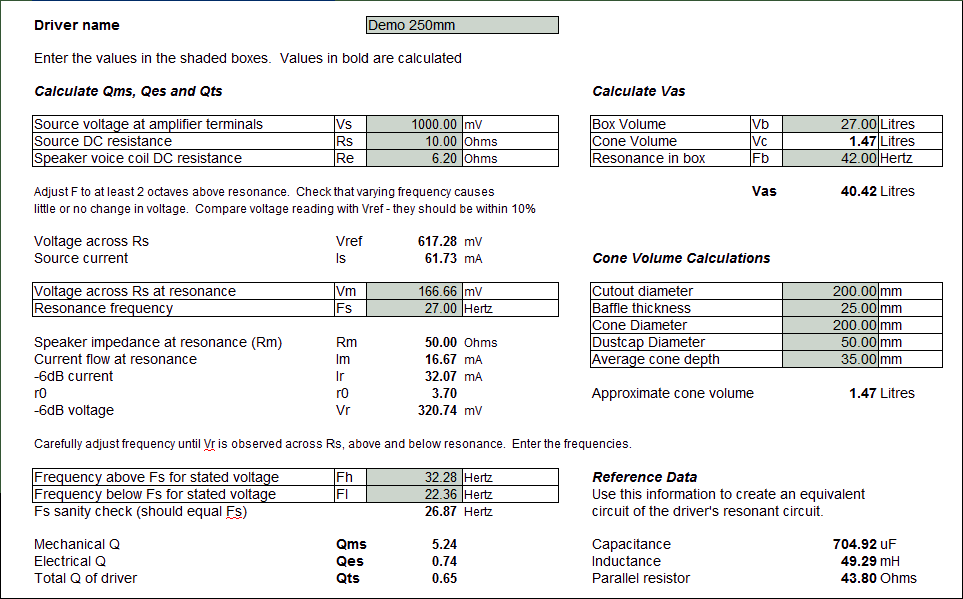Figure 6 - Test Box Calculation Example Using Spreadsheet

As you can see from the screen-shot, the spreadsheet will calculate everything for you, including the cone volume, Vas and the values shown in the schematic of the driver.  Naturally, you will get figures quite different from those shown, but the principle is exactly the same.

3   Measuring Vas (equivalent air compliance), Method 2

The second method is to use an added mass, M1.  Typically modelling clay or Blu-Tak is simply stuck to the cone close to the voicecoil, and the change of resonant frequency allows you to determine the moving mass of the cone.  Armed with this, you can then calculate the Vas.

For speakers less than 200mm (8"), use 5 grams, for 200mm use 10g, and for 250mm (10") or larger, use 20g.  You may need to add more if the mass chosen does not reduce resonance by at least 10%.  The mass must be measured accurately! Even a small error can cause a large variation in the calculated Vas, so a precision scale (accurate to at least 0.1g) is essential.

You also need to measure the effective cone diameter.  This is generally taken as a measurement that includes half the surround.  Again, an inaccurate reading will make a big difference.  Because of this, the test box method is probably more accurate.  You don't need to worry about extremely accurate measurements that have a profound effect on the measurement result.  Still, the added mass method is quick and convenient, and many people (including me) find it quicker and easier than using a known volume.

The Fs of the speaker in free air has been measured, so simply add a suitable mass to the cone and re-measure the resonant frequency.  This becomes Fs¹.

First, measure cone diameter so that effective cone area can be determined.  Measure the diameter, including half the surround.  The measurement must be in centimetres for this calculation.  Divide by 2 to get the radius ...

A = π × r²

Calculate cone mass ...

M = M1 / (( Fs / Fs¹ )² -1 )

Next, determine Cms ...

Cms = 1 / ( 2 × π × fs )² × M
Vas = Cms × d × c² × A²

Assume the following ...

d = density of air = 0.001204 g/ml
c = speed of sound = 345 m/s       (24°C with ~50% humidity, or 343 m/s at 20°C)

Let's do a sample calculation using the same driver as before.  The downloadable spreadsheet includes both methods, enabling a direct comparison if you use the two different calculations.  Everything stays the same, but we no longer have to use a reference box.  We also don't need to determine the cone volume, only the area.  As a matter of course, I generally use 345m/s as the speed of sound, which allows for a more realistic temperature with Australian conditions.  It can be determined for any temperature with the following formula ...

c = 331.4 + ( 0.6 × t c )     (Where t c is the temperature of air in °C)    (HyperPhysics)

For the sake of this exercise, we measure the cone diameter and obtain 200mm, including half the surround.  Divide the diameter in millimetres by 20 to obtain the radius in centimetres ...

A = π × r²     = π × 10²     = 314.16 cm²

Next, we measure the driver's resonance with an added mass.  The mass was carefully measured, and was 45.80 grams (note that it was indicated above that some drivers will need a lot more mass than may be indicated - this is just such a driver, because it has a heavy cone) ...

M = M1 / (( Fs / Fs¹ )² -1 )     = 45.8 / (( 27 / 23 )² -1)     = 45.8 / ( 1.378 - 1) = 45.8 / 0.378 = 121.16 grams

Now we can calculate Cms, using the values shown above ...

Cms = 1 / (( 2 × π × fs )² ) × M     = 1 / ( 2 × π × 27 )² × 121.14     = 1 / ( 169.64² × 121.14 ) = 2.87E-7

Now that we have everything needed, Vas can be calculated, using the default values for air density and sound velocity ...

Vas = Cms × d × c² × A² × 10    = 2.87E-7 × 0.001204 × 345² × 314.16² × 10    = 40.57 litres

Note: the ×10 was added to make corrections for different units being used (e.g. m/s for velocity, litres, millilitres, cm², etc.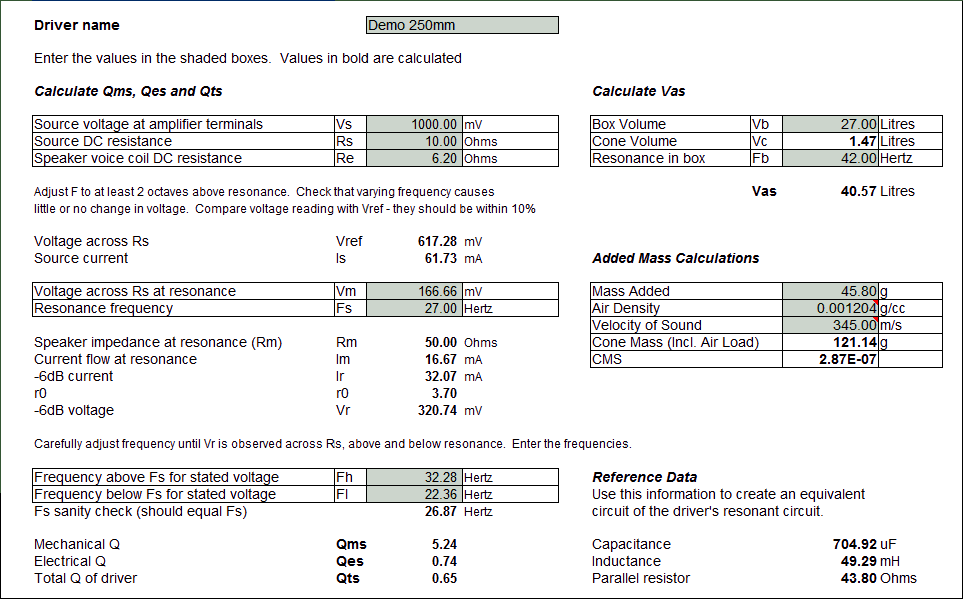Figure 7 - Added Mass Calculation Example Using Spreadsheet

The spreadsheet may give a slightly different answer because it calculates all values to the maximum number of decimal places.  The values shown here are limited to two decimal places for clarity.  Note that 2 cells (indicated by a small red triangle) have comments attached.  The comment will show when your mouse pointer is over the cell.  Please read before you go changing anything.  All driver initial measurements are imported from the 'known volume' sheet, and do not need to be re-entered.

It must be pointed out that the (imaginary) driver used for the demonstration calculations is rather unlikely, so don't expect to get even remotely similar figures.  A 250mm driver with a cone weighing in at almost 122 grams would certainly have a low resonance, but would also be pathetically inefficient.  However, the important thing here is to show the principles involved and the methods of calculation.  Even bass drivers will usually have a lighter cone, so the Vas will be much larger than these demonstration calculations might imply.  It's a sad fact of life, but loudspeakers continue to be riddled with compromise.  For high efficiency you need a light cone, and a light cone means a large Vas which in turn requires a large enclosure if you actually want to get bass from it.

4   Calculate Speaker Equivalent Circuit

To determine the equivalent circuit of the speaker, we need to look at its behaviour at resonance.  Rp is the apparent resistance in parallel with the resonant circuit, consisting of Cr (resonance capacitance) and Lr (resonance inductance).

Rp = Rm - Re
Cr = 1 / ( 2 × π × ( Rp / Qms ) × Fs ) µF
Lr = ( Rp / Qms ) / (2 × π × Fs ) mH

For the speaker shown, these values are the same as shown in the spreadsheet.  Calculating the voicecoil's semi-inductance requires that another measurement be taken, to examine the impedance at higher frequencies.  If you measure impedance at (say) 2kHz (19.7 ohms) and 4kHz (38.2 ohms), the inductance can be determined ...

L = Δ Z / ( 2 × π × Δ f )       (Where Δ Z is impedance change and Δ f is frequency change)
L = 18.5 / ( 2 × π × 2kHz ) = 1.47mH

This will never be particularly accurate because the inductance is lossy (due to eddy currents in the pole pieces), but it's not a bad place to start if you want to build a simulation of your speaker.

Reference
My thanks to Brian Steele for allowing me to use the simplified method and formulae he devised for Thiele/Small parameter measurement, and also Vas measurement information.  Brian's original data are available Here.  My thanks also to Jay Taylor for corrections and an updated spreadsheet that managed to get the calculations right.

The added mass method is from "How to Design, Build, & Test Complete Speaker Systems", by David Weems, published by TAB Books, 1978

Thanks to Michael T. for pointing out a discrepancy between Neville Thiele's Qes calculation and the one that was originally used here.  The formula used was that from Theile, but it's since been found to provide a Qes value that is much too high.  The original is now used instead.  The formula has been changed back to the one used from the outset both in this article and in the spreadsheet.Main IndexArticles Index